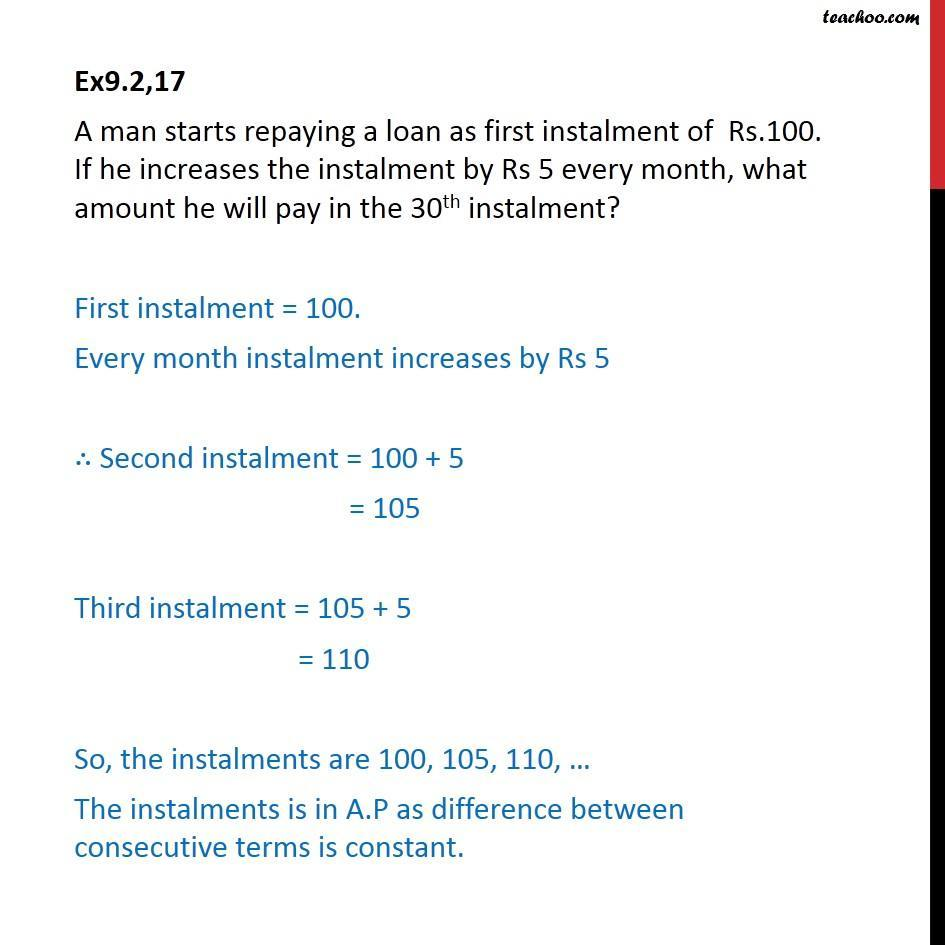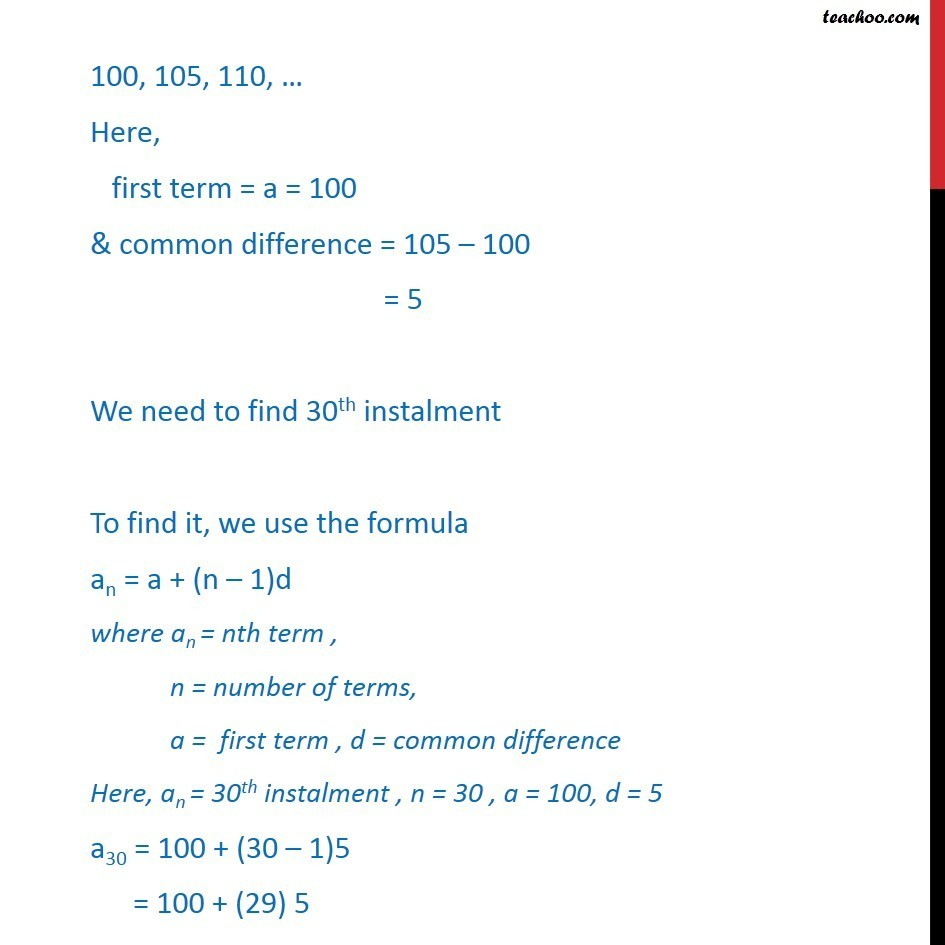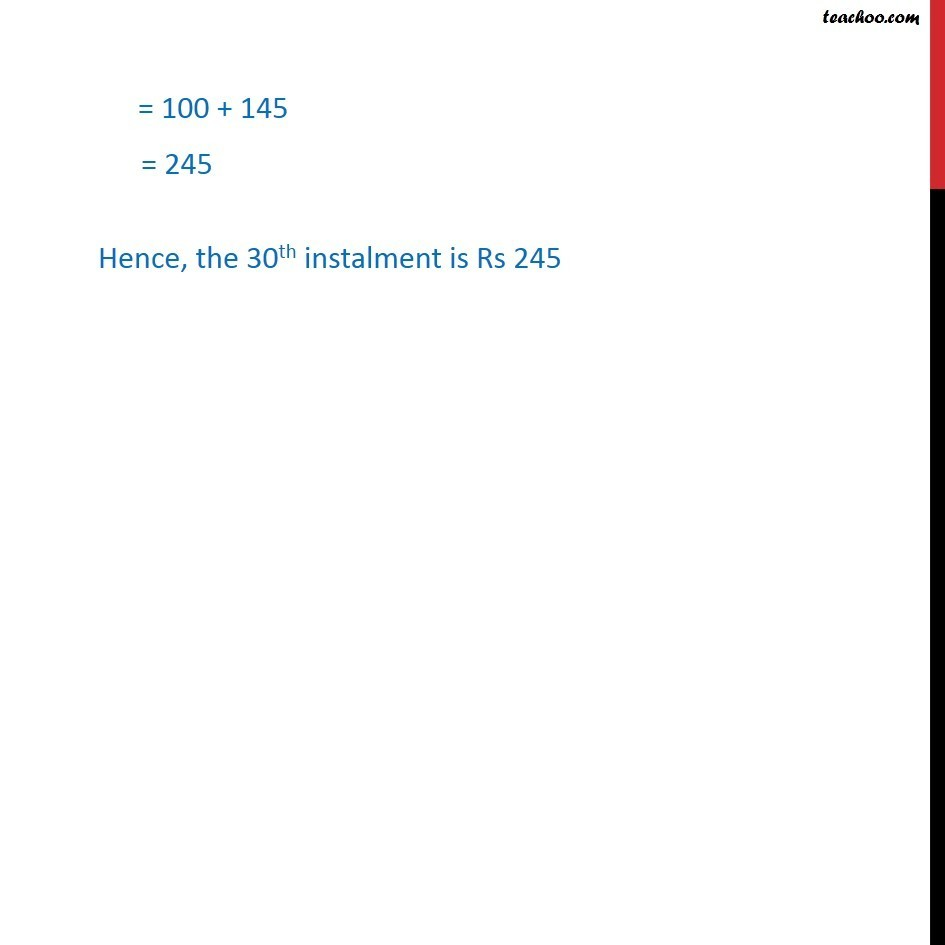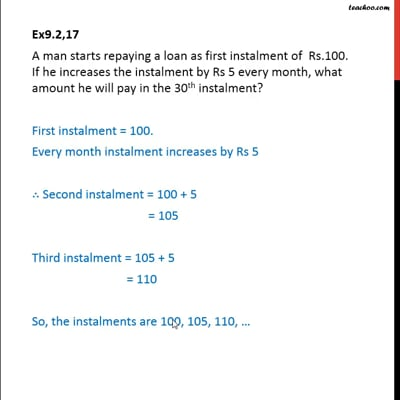Arithmetic Progression (AP): Statement

Chapter 9 Class 11 Sequences and Series
Concept wiseThis video is only available for Teachoo black users

Introducing your new favourite teacher - Teachoo Black, at only ₹83 per month

### Transcript

Ex9.2,17 A man starts repaying a loan as first instalment of Rs.100. If he increases the instalment by Rs 5 every month, what amount he will pay in the 30th instalment? First instalment = 100. Every month instalment increases by Rs 5 Second instalment = 100 + 5 = 105 Third instalment = 105 + 5 = 110 So, the instalments are 100, 105, 110, The instalments is in A.P as difference between consecutive terms is constant. 100, 105, 110, Here, first term = a = 100 & common difference = 105 100 = 5 We need to find 30th instalment To find it, we use the formula an = a + (n 1)d where an = nth term , n = number of terms, a = first term , d = common difference Here, an = 30th instalment , n = 30 , a = 100, d = 5 a30 = 100 + (30 1)5 = 100 + (29) 5 = 100 + 145 = 245 Hence, the 30th instalment is Rs 245